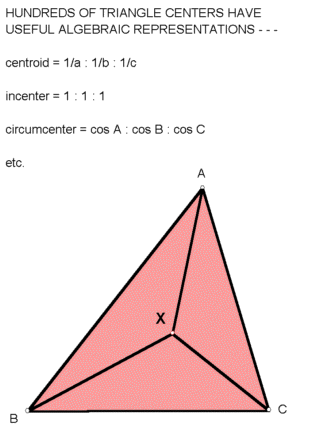# ALGEBRAIC HIGHWAYS

## IN TRIANGLE GEOMETRY

 Coordinates, expressed in terms of angles A,B,C (or sidelengths a,b,c) of a variable triangle ABC, enable highways in triangle geometry that are much more algebraic than most of those traveled during earlier times. Certain notions and notations are prerequisite, especially trilinear coordinates.) These are often called simply trilinears. Using these, we have a very basic algebraic definition: DEFINITION OF TRIANGLE CENTER Suppose a point P has a trilinear representation f(A,B,C) : g(A,B,C) : h(A,B,C) such that (i) g(A,B,C ) = f(B,C,A)and h(A,B,C) = f(C,A,B); (ii) f(A,C,B) = f(A,B,C); (iii) if P is written as u(a,b,c) : u(b,c,a) : u(c,a,b), where a,b,c are the sidelengths of triangle ABC, then u is homogeneous in the variables a,b,c. (By the law of sines and (i), such u must exist.) Then P is a triangle center, or simply a center.Example 1: 1/(b-c) : 1/(c-a) : 1/(a-b) is a center, since it equals

f(A,B,C) : f(B,C,A) : f( C,A,B),
where f(A,B,C) = (sin C - sin A)(sin B - sin A).

More examples: 1 : 1 : 1 (incenter),
csc A : csc B : csc C (centroid),
cos A : cos B : cos C (circumcenter),
sec A : sec B : sec C (orthocenter),
csc(A + pi/3) : csc(B + pi/3) : csc(C + pi/3) (Fermat point).

MAJOR CENTERS

A triangle center is a major center if it has a trilinear representation of the form f(A) : f(B): f(C). The five centers mentioned above are major centers. In

C. Kimberling, "Major centers of triangles," Amer. Math. Monthly 104 (1997) 431-438,

a construction is given for the major center x(A) : x(B) : x(C), where x(A) = f(A/2)sec(A/2), and subsequent constructions and limit-constructions, including the bare-angle center, A : B : C. If triangle ABC is acute, the cited reference gives a construction for the major center y(A) : y(B) : y(C), where y(A) = (csc 2A)/f(pi/2 - A). Since y(y(A)) = f(A), the mapping y could be regarded as a (new) kind of conjugate, if someone could find a satisfactory way to extend y to obtuse triangles. (If you succeed, let me know.)

Other major centers derived from any given major center are discussed in

C. Kimberling, "A class of major centers of triangles", Aequationes Mathematicae, forthcoming.

CONSTRUCTIVE SYSTEMS

Suppose you have a set of rules for constructing objects (e.g., points and lines) and an initial set of objects - then you also have the set of all objects thereby constructible. If you now introduce a new rule, you have a new set of constructible objects. In the study cited below, each rule is called an opus, and the set objects constructible under a specified set of opera (the plural of opus) is called a constructive system. The basic questions are, what minimal sets of opera are needed to generate various objects of interest, and how many "steps" are necessary? In

C. Kimberling, "Constructive systems in triangle geometry", Nieuw Archief voor Wiskunde 15 (1997) 163-173,

the primary system is generated by four primary opera, defined for points P, Q, lines K,L, and the line LL at infinity, as follows:

opus 1: line PQ if P<>Q,
opus 2: point (K intersect L) if K not = L,
opus 3: line through P parallel to K, except if K=LL,
opus 4: line through P perpendicular to K, except if K=LL.

Repeated applications of the four opera to the set {A,B,C} generate the primary system, which contains, for example, the centroid, circumcenter, orthocenter, and Euler line, but not the Fermat point or incenter.

One can, for example, adjoin to the initial four opera any subset of the following three additional opera, expressed in terms of points P,Q not on LL, line L (not LL), and circles U,V :

opus 5: the circle having segment PQ as diameter,
opus 6: the point (L intersect U) if L is tangent to U, else the two points,
opus 7: the point (U intersect V) if U is tangent to V, else the two points.

PRODUCTS, SQUARE ROOTS, AND LAYERS

Suppose points P and Q in the plane of a triangle ABC are given by trilinears:

P = u : v : w and Q = x : y : z.

Cyril Parry has devised simple constructions for products and square roots of points in the plane of a triangle ABC, these being defined by

P*Q = ux : vy : wz and SQRT(P) = sqrt(u) : sqrt(v) : sqrt(w).

These constructions will appear in

C. Kimberling and C. Parry, "Products, square roots, and layers in triangle geometry", in progress.

A summary-introduction of "layers" is not attempted here, but some hint about "n-layers" can be gleaned from the Conclusion of the above cited paper: ". . . we can now [systematically] construct any finite product of any integer powers of any points of the forms

sin kA : sin kB : sin kC,
cos mA : cos mB : cos mC,
cos(nB - nC) : cos(nC - nA) : cos(nA - nB);

moreover, whenever such a product lies not ouside triangle ABC, we can construct its square root . . .